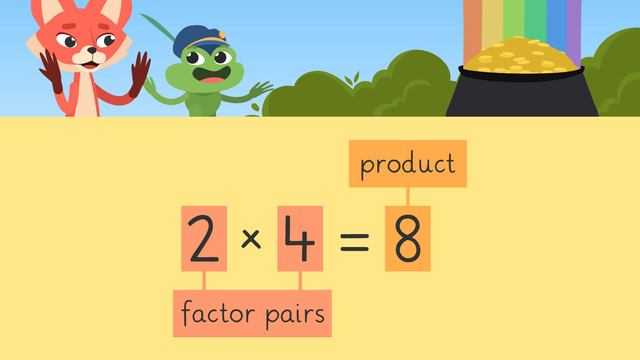# Factors / Factor Pairs

Content Factors / Factor PairsRating

Ø 5.0 / 1 ratings
The authorsTeam Digital
Factors / Factor Pairs
CCSS.MATH.CONTENT.4.OA.B.4

## Factor Pairs

### TranscriptFactors / Factor Pairs

Skylar and Henry found a pot of gold at the end of a rainbow, but it is guarded by a cunning troll. He demands that they find all the numbers that divide into seventy-eight evenly before they can take it. But first, Skylar and Henry must learn about factors and factor pairs. A factor is a number that can be divided into a whole number evenly. Because multiplication is the inverse of division, we can say that a factor multiplied by another factor equals a product. Factor pairs are the two numbers we multiply together to get the product. In this number sentence, the factor pair of eight is two and four. But these are not the only factor pairs that equal eight. We know that we can get any whole number by multiplying one by the number itself, so one and eight are also a factor pair of eight. From our sets of factor pairs, we can list the factors for a number. The factors of eight are one, two, four, and eight. When finding all the factor pairs of a number, it helps to use a system. One system is called the "rainbow method." The "rainbow method" uses counting numbers and divisibility. Divisibility means it can be divided evenly by another number. Let's use this system to find the factors of eighteen. To start, put the first counting number, "one," and the partner factor, "eighteen," here. Now, write the next counting number, two... and think: what number do we multiply two by to make eighteen? (...) Nine. What about three? (...) Three times six makes eighteen. Now, try four. Can we multiply four by a number to get eighteen? (...) No, four is not a factor of eighteen. Neither is "five." We can see that "six" is already paired on this side of the rainbow, so we have found all the factor pairs. Now we list them in order. The factors of eighteen are one, two, three, six, nine, and eighteen. Let’s try the number forty. We have one and (...) forty,... and two and (...)twenty. What is the next number that would be a factor of forty? (...) Four. What is the partner factor for four? (...) Ten. What is the next set of factor pairs? (...) five and (...) eight. Is six a factor of forty? (...) No. How about seven? (...) No, forty is not divisible by either number. Eight is already on this side of the chart, ... so we have found all of the factor pairs for forty. Now, list the factors in order… One, two, four, five, eight, ten, twenty, and forty Here’s one to try on your own. Find all the factor pairs of seventy-eight and then list the factors in order. Remember, to find the pairs, go through all the counting numbers and check for divisibility. Pause the video to solve, and press play when you are ready to continue. The factor pairs of seventy-eight are... one and (...) seventy-eight; two and (...) thirty-nine; three and (...) twenty-six; and six and (...) thirteen. The factor pairs of seventy-eight are... and we list all the factors in order like this. Remember, a factor is a number that can be divided into a whole number evenly. Because multiplication is the inverse of division, we can say that a factor multiplied by another factor equals a product. Factor pairs are the two numbers we multiply together to get the product. And with that, the troll gave a wave and disappeared. “We’re RICH!” “It’s (...) it’s (...) it’s CHOCOLATE!” “Mmmm, rich dark chocolate!”